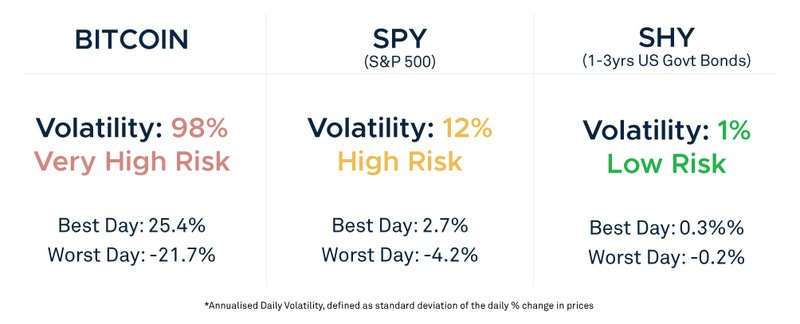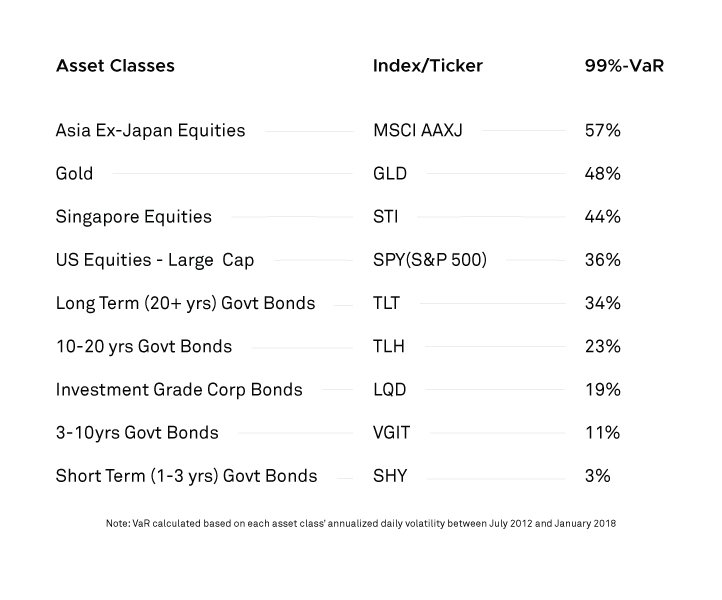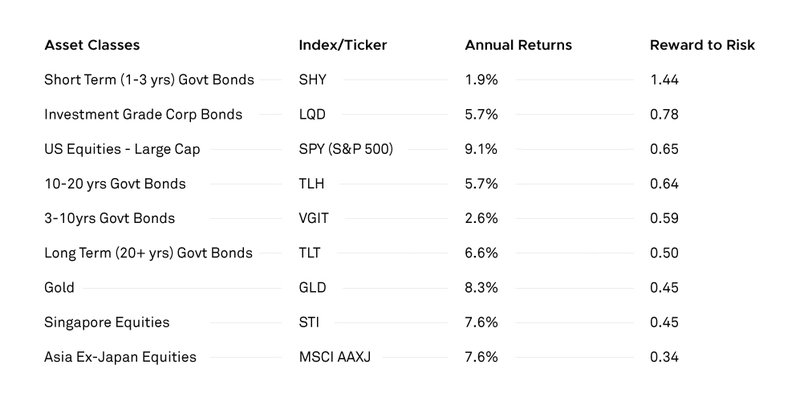# Debunking "High Risk, High Return"

Is 6% annual returns high or low? It depends!

How much risk was taken to deliver that 6% in returns? If you’re comparing returns of different investment products, you should be looking at how much risk it took to achieve those returns. We all know the saying, “High Risk, High Returns”, but how true is it?

The short answer is: in the long-term, on average, riskier investments will probably give higher returns. The key words in that sentence are “long-term” and “average”. In the short term, riskier investments are more likely to give lower returns and experience more losses.

Here, I’m going to focus on how to understand risk and holistically interpret returns.

## Understanding risk

In investing, risk is often measured by volatility. A more volatile investment is riskier than a less volatile investment. Volatility can be observed by the daily or weekly swings in price; the larger swings in price, the greater the volatility.

In Table 1, you can easily see the difference between a very high-risk asset class (Bitcoin), a high-risk asset class (SPY, an ETF indexed to the S&P500), and a low-risk asset class (SHY, an ETF indexed to 1-3 years US Government Bonds).

#### Table 1: Daily and Weekly Volatility of Bitcoin, SPY and SHY from 1 July 2017 to 30 June 2018Between July 2017 and July 2018, Bitcoin had a week where a \$100,000 USD investment would have become \$170,000 USD by end of the week (\$70,000 USD gain), and also a week where a \$100,000 USD investment would have become \$62,000 USD by end of the week, with a \$38,000 USD loss. Obviously, this is an incredibly risky investment. On the other end of the spectrum, in its best week, Short Term US Government Bonds has turned a \$100,000 USD investment in \$100,500 USD (\$500 USD gain) and in the worst week of the last 12 months has turned a \$100,000 USD investment in \$99,600 USD (\$400 USD loss).

Bitcoin had 8x the volatility of the equity market, and equities showed 12x the volatility of short-term government bonds.

## Understanding volatility

A common way to compare the risk of different investment options and make volatility a more tangible measure is to look at Value at Risk (VaR). VaR refers to the probability that an investor can expect to lose a given percentage of his or her investments in a given year. VaR is expressed as a percentage that refers to the probability of losing a given percentage of an investment. So, a 99%-VaR indicates that an investor has a 99% chance of not losing the given percentage of his or her investments, and an 80%-VaR indicates that an investor has an 80% chance of not losing the given percentage of his or her investments. Now that we understand that the %-VaR refers to the probability of losing a percent of your money, we then need to understand how much money you would expect to lose in that probable event. To illustrate, StashAway calculates that with 99%-VaR that Portfolio 1 has a VaR of 2%. This should be interpreted that there is a 99% chance that you won’t lose 2% of your investments, or, there is a 1% chance that you will lose 2% of your investments.

VaR makes it easy to compare the riskiness of different portfolios or individual asset classes. For example, the STI index has a 99%-VaR of 44%, and therefore an investment in the STI ETF carries a 1% probability of losing 44% of the money in a given year; bonds are less risky, and in fact, investing in a basket of investment grade corporate bonds carries a 1% probability of losing 19% of the money in a given year.

To illustrate how much different asset classes vary in Value at Risk, the following table summarises 99%-VaR for a few selected asset classes, ranked from riskier to less risky.

### Table 2. Asset Classes Compared by 99%-Value at RiskNote: VaR calculated based on each asset class’ annualised daily volatility between July 2012 and January 2018

## Measuring risk

There are many different ways and different ratios that professional investors look at, including Sharpe Ratio and Sortino Ratio. One effective and simple ratio is “Reward to Risk”, or the relationship between average annual returns and annualised daily volatility: an investment giving 5% returns with a 5% volatility has a Reward to Risk ratio of 1.0, which makes its risk-adjusted returns better than an investment giving 8% returns with 20% volatility (Reward to Risk = 8/20 = 0.4).

The following table summarises average yearly returns and Reward to Risk ratio of a few asset classes between July 2012 and January 2018, ranked by “Reward to Risk” ratio.

### Table 3. Asset Classes Compared by Reward to Risk RatioOver the last 15 years, US equities have provided better risk-adjusted returns than Singaporean and Asian equities, and bonds have performed well. So while the 7.6% returns per annum of the STI are good average returns over 15 years, it is important to note that similar or higher returns could have been achieved taking lower risk.

## In short, don’t take unnecessary risk

In investing, you cannot talk about returns without talking about risk, and you should never invest in a product based on its historical and projected returns, without having first learnt about its volatility and overall risk. Make sure you do your homework before deciding on an investment product.